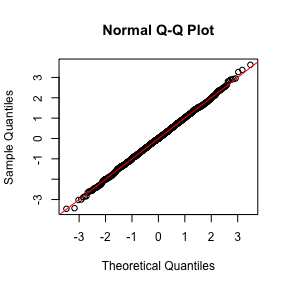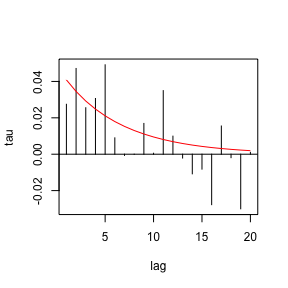# Basic Time Series Copula Processes

#### 2022-05-07

This library currently implements 3 basic kinds of time series copula process: ARMA copula processes and d-vine copula processes of type 1 and type 2. These are described in the next 3 sections.

## 1. ARMA Copula Processes

### AR(1) Example

An ARMA copula is specified by a list of two vectors, the first named ar and the second ma. The following example creates an AR(1) copula process specification and then displays the spec.

ar1 <- armacopula(list(ar = 0.7))
ar1
#> object class: armacopula
#> name: ARMA(1,0)
#> parameters:
#> ar1
#> 0.7

A realization can be generated with the generic command sim.

set.seed(13)
data1 <- sim(ar1, 1000)
ts.plot(data1)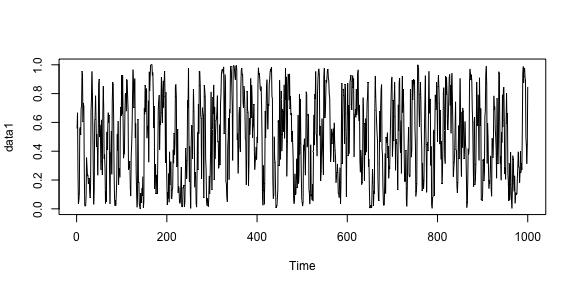A model specification can be fitted to data with the generic command fit.

ar1spec <- armacopula(list(ar = 0))
ar1fit <- fit(ar1spec, data1)
ar1fit
#> object class: armacopula
#> name: ARMA(1,0)
#> _____________________
#> Summary of estimates:
#>    ar.ar1
#> 0.7041692
#> convergence status: 0, log-likelihood: 352.1703

### ARMA(1,1) Example

The next example simulates and fits an ARMA(1,1) copula process, giving standard errors for the parameter estimates.

arma11 <- armacopula(list(ar = 0.95, ma = -0.85))
data2 <- sim(arma11, 1000)
ts.plot(data2)

arma11spec <- armacopula(list(ar = 0.1, ma = 0.1))
arma11fit <- fit(arma11spec, data2, tsoptions = list(hessian = TRUE))
arma11fit
#> object class: armacopula
#> name: ARMA(1,1)
#> _____________________
#> Summary of estimates:
#>         ar.ar1      ma.ma1
#> par 0.95266496 -0.86527336
#> se  0.01778232  0.02830486
#> convergence status: 0, log-likelihood: 32.68804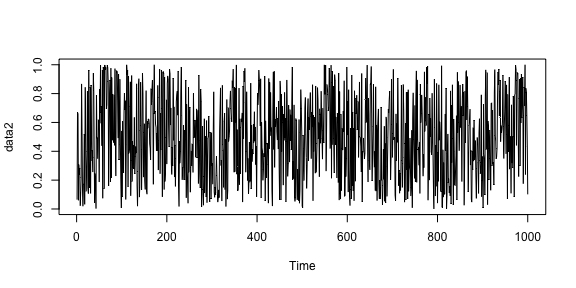Coefficients of the fitted model are obtained with the command coef, residuals with the command resid and various plots are generated by the generic command plot.

coef(arma11fit)
#>        ar1        ma1
#>  0.9526650 -0.8652734
res <- resid(arma11fit)
acf(res)
acf(abs(res))
plot(arma11fit)
plot(arma11fit, plottype = "kendall")
mu_t <- resid(arma11fit, trace = TRUE)
ts.plot(mu_t)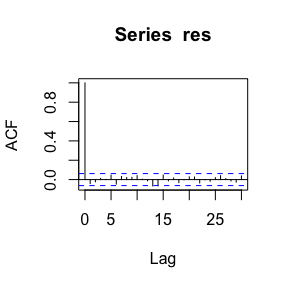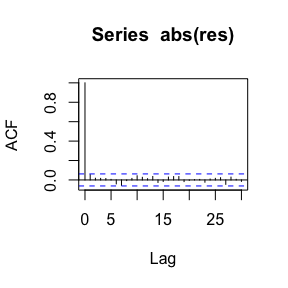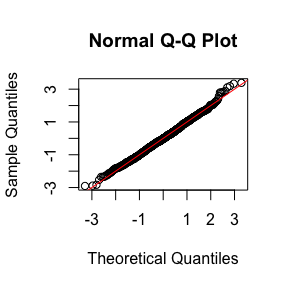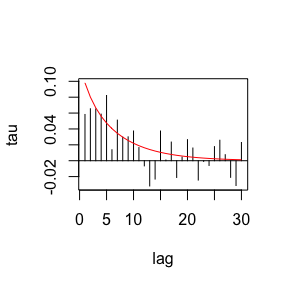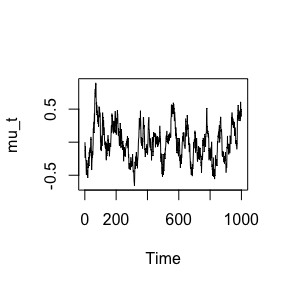The data for these plots come from applying the Kalman filter command kfilter.

head(kfilter(arma11fit@tscopula, data2))
#>             mu_t   sigma_t      resid
#> [1,]  0.00000000 1.0000000 -1.5175954
#> [2,] -0.23284612 0.9881594  0.6746350
#> [3,] -0.13131359 0.9803361  0.5419625
#> [4,] -0.05949498 0.9749751 -1.4900299
#> [5,] -0.22337772 0.9712090 -0.2815984
#> [6,] -0.24246851 0.9685171 -0.4745894

## 2. D-Vine Copula Processes (type 1)

### Construction

We construct a copula of order $$p = 3$$ in which the copulas are respectively Clayton, Frank, and Gauss. The parameters are given in a list. Individual copulas can be rotated through 180 degrees.

copmod <- dvinecopula(
family = c("Clayton","Frank", "Gaussian"),
pars = list(1.2, 2, 0.15),
rotation = c(180,0, 0)
)
copmod
#> object class: dvinecopula
#> name: d-vine(3)
#> copula family:  clayton180 frank gaussian
#> parameters:
#> cop1.p1 cop2.p1 cop3.p1
#>    1.20    2.00    0.15

### Simulation

A realization can be generated with the generic command sim.

set.seed(29)
data1 <- sim(copmod, n = 2000)
hist(data1)
ts.plot(data1)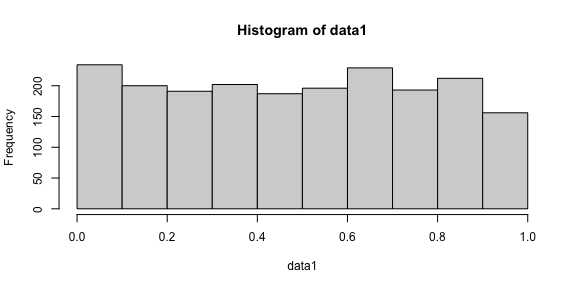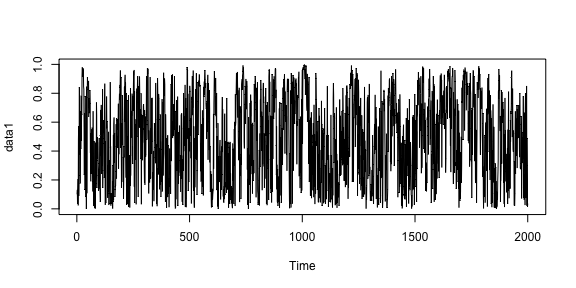### Estimation

A model spec can be fitted to data with the generic command fit.

copspec <- dvinecopula(
family = c("Clayton","Frank", "Gaussian"),
pars = list(0.5, 1, 0),
rotation = c(180, 0, 0)
)
copfit <- fit(copspec, data1,
tsoptions = list(hessian = TRUE),
control = list(maxit = 2000))
copfit
#> object class: dvinecopula
#> name: d-vine(3)
#> copula family:  clayton180 frank gaussian
#> _____________________
#> Summary of estimates:
#>        cop1.p1   cop2.p1    cop3.p1
#> par 1.06144147 1.9009764 0.15965799
#> se  0.05300061 0.1252102 0.02115241
#> convergence status: 0, log-likelihood: 448.3769
coef(copfit)
#>  cop1.p1  cop2.p1  cop3.p1
#> 1.061441 1.900976 0.159658
coef(copmod)
#> cop1.p1 cop2.p1 cop3.p1
#>    1.20    2.00    0.15

### Plotting

Various plots are generated by the generic command plot.

plot(copfit)
plot(copfit, plottype = "kendall")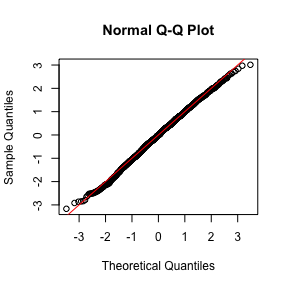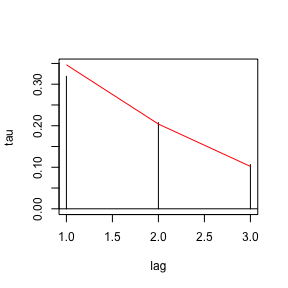Here is the generalized lag plot.

plot(copfit, plottype = "glag")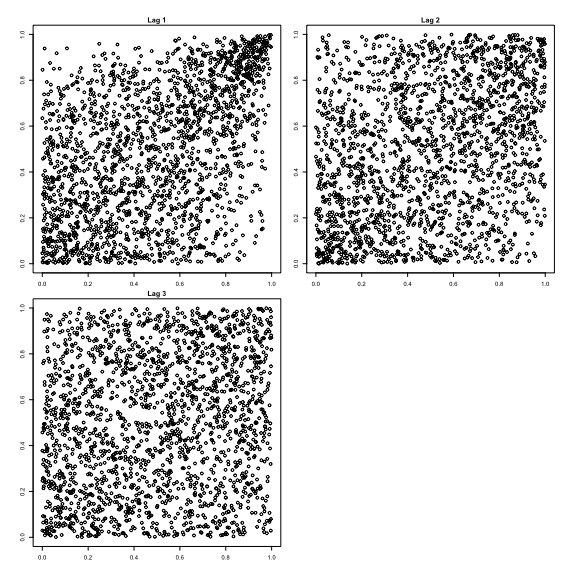## 3. D-Vine Copula Processes (type 2)

### Construction

We construct a model using the Joe copula and the Kendall partial autocorrelation function (KPACF) of a Gaussian ARMA process with autogressive (ar) parameter 0.9 and moving average (ma) parameter -0.85. The KPACF is truncated at lag 20, so this is a process of finite order. We can also set $$\text{maxlag} = \inf$$ but this leads to much slower simulation.

copmod <- dvinecopula2(family = "joe",
kpacf = "kpacf_arma",
pars = list(ar = 0.9, ma = -0.85),
maxlag = 20)
copmod
#> object class: dvinecopula2
#> name: type2-d-vine
#> copula family: joe
#> KPACF: kpacf_arma with max lag 20
#> parameters:
#>    ar    ma
#>  0.90 -0.85

### Simulation

A realization can be generated with the generic command sim.

set.seed(13)
data1 <- sim(copmod, n = 2000)
hist(data1)
ts.plot(data1)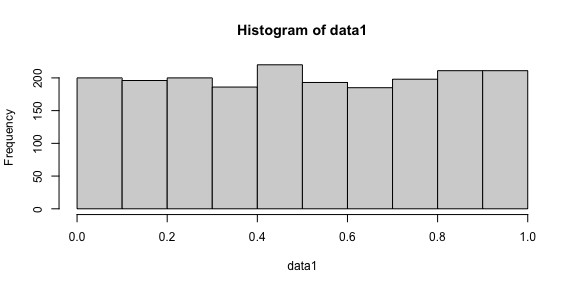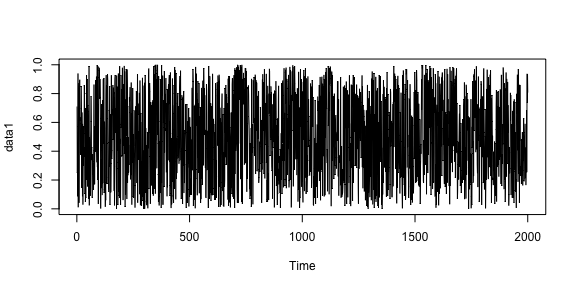### Estimation

A model spec can be fitted to data with the generic command fit.

copspec_Gauss <- dvinecopula2(family = "gauss",
pars = list(ar = 0, ma = 0),
maxlag = 20)
fitGauss <- fit(copspec_Gauss, data1)
fitGauss
#> object class: dvinecopula2
#> name: type2-d-vine
#> copula family: gauss
#> KPACF: kpacf_arma with max lag 20
#> _____________________
#> Summary of estimates:
#>         ar         ma
#>  0.9234119 -0.8695245
#> convergence status: 0, log-likelihood: 21.26753

copspec_Joe <- dvinecopula2(family = "joe",
pars = list(ar = 0, ma = 0),
maxlag = 20)
fitJoe <- fit(copspec_Joe, data1)
fitJoe
#> object class: dvinecopula2
#> name: type2-d-vine
#> copula family: joe
#> KPACF: kpacf_arma with max lag 20
#> _____________________
#> Summary of estimates:
#>         ar         ma
#>  0.9052847 -0.8535974
#> convergence status: 0, log-likelihood: 48.98484

AIC(fitGauss, fitJoe)
#>          df       AIC
#> fitGauss  2 -38.53506
#> fitJoe    2 -93.96967

### Plotting

Various plots are generated by the generic command plot.

plot(fitJoe)
plot(fitJoe, plottype = "kendall")